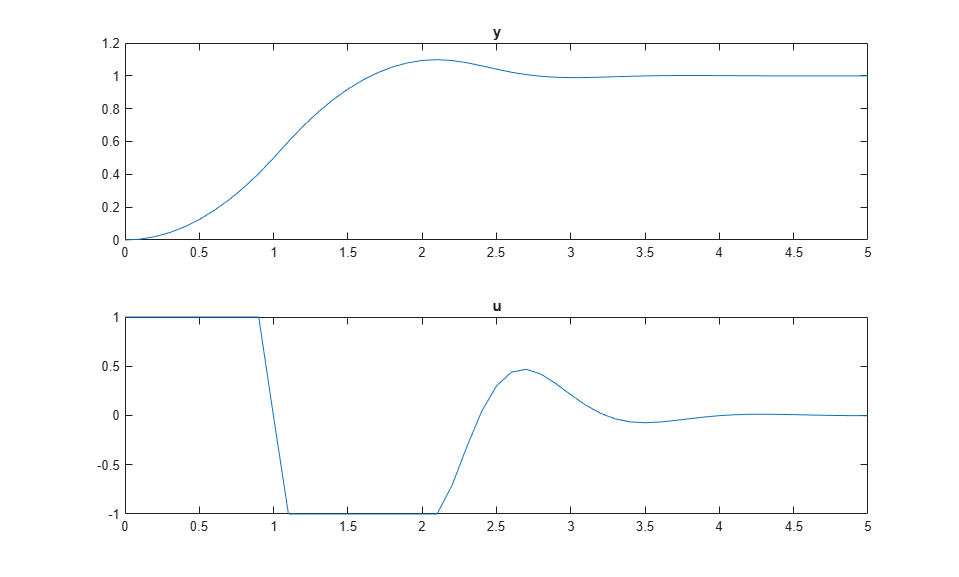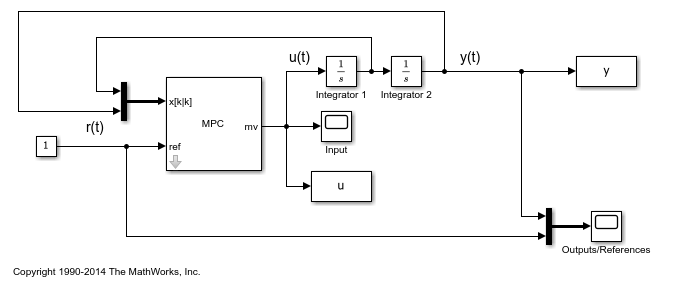Documentation

### This is machine translation

Mouseover text to see original. Click the button below to return to the English version of the page.

## Using Custom State Estimation

This example shows how to use measurable plant states in MPC control at run time.

### Define Plant Model

The linear open-loop plant model is a double integrator.

```plant = tf(1,[1 0 0]); ```

### Design MPC Controller

Create the controller object with sampling period, prediction and control horizons.

```Ts = 0.1; p = 10; m = 3; mpcobj = mpc(plant, Ts, p, m); ```
```-->The "Weights.ManipulatedVariables" property of "mpc" object is empty. Assuming default 0.00000. -->The "Weights.ManipulatedVariablesRate" property of "mpc" object is empty. Assuming default 0.10000. -->The "Weights.OutputVariables" property of "mpc" object is empty. Assuming default 1.00000. ```

Specify actuator saturation limits as MV constraints.

```mpcobj.MV = struct('Min',-1,'Max',1); ```

Specify the controller to use custom state estimation.

```setEstimator(mpcobj,'custom'); ```

### Simulate Using MPCMOVE Command

Configure variables to store the closed-loop responses.

```Tf = round(5/Ts); YY = zeros(Tf,1); UU = zeros(Tf,1); ```

Prepare the plant used in simulation.

```sys = c2d(ss(plant),Ts); xsys = [0;0]; ```

Use MPCSTATE object to specify the initial controller states before simulation starts.

```xmpc = mpcstate(mpcobj); ```
```-->Converting the "Model.Plant" property of "mpc" object to state-space. -->Converting model to discrete time. Assuming no disturbance added to measured output channel #1. -->The "Model.Noise" property of the "mpc" object is empty. Assuming white noise on each measured output channel. ```

Simulate closed-loop response iteratively.

```for t = 0:Tf % Update plant measurement. ysys = sys.C*xsys; % Compute MPC action. Because the plant states are measurable, use % these values for state estimation instead of the plant output. xmpc.Plant = xsys; u = mpcmove(mpcobj,xmpc,[],1); % Store signals. YY(t+1) = ysys; UU(t+1) = u; % Update plant states. xsys = sys.A*xsys + sys.B*u; end subplot(2,1,1) plot(0:Ts:5,YY); title('y'); subplot(2,1,2) plot(0:Ts:5,UU); title('u'); ```To run this example, Simulink® is required.

```if ~mpcchecktoolboxinstalled('simulink') disp('Simulink(R) is required to run this example.') return end ```

Simulate closed-loop control of the linear plant model in Simulink. Controller "mpcobj" is specified in the MPC Controller block dialog.

```mdl = 'mpc_customestimation'; open_system(mdl); sim(mdl); ```The closed-loop responses are identical between simulations in MATLAB and Simulink.

```fprintf('\nDifference between simulations in MATLAB and Simulink is %g\n',norm(UU-u)); ```
```Difference between simulations in MATLAB and Simulink is 7.66845e-14 ```
```bdclose(mdl) ```